MORE IN Applied Mathematics 4
MU Mechanical Engineering (Semester 4)
Applied Mathematics 4
December 2013
Total marks: --
Total time: --
INSTRUCTIONS
(1) Assume appropriate data and state your reasons
(2) Marks are given to the right of every question
(3) Draw neat diagrams wherever necessary

1 (a) Find the fourier expansion of f(x) = 4 - x2 in the interval (0,2)
5 M
1 (b) Find the probability that at most 5 defective fuses will be found in a box of 200 fuses if experience shows that 2% of such fuses are defective.
5 M
1 (c) Given 6y = 5x + 90, 15x = 8y + 130, (σx)2 = 16. Find :
(i) ¯x and ¯y
(ii) r
(iii) (σy)2
5 M
1 (d)

Solve the two dimensional heat equation$$\dfrac{d^{2}u}{dx^{2}}+\dfrac{d^{2}u}{dy^2}=0$$ which satisfies the conditions u(0, y)=u(l, y)=u(x, 0) and u(x, a) = sin $$\dfrac{n \pi x}{l}$$

5 M

2 (a) Obtain fourier series for f(x) = x - x2, -π < x < π. Hence deduce that: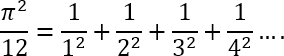7 M
2 (b) Seven dice are thrown 729 times. How many times do you expect atleast 4 dices to show three or five?
7 M
2 (c) A continuous random variable X had pdf f(x) = kx2 e-x, x ≥ 0.Find k mean and variance.
6 M

3 (a) Using normal distribution find the probability that in a group of 100 persons there will be 55 males assuming that the probability of a person being male is 1/2
7 M
3 (b) Derive wave equation for vibration of string.
7 M
3 (c) Obtain fourier expansion of f(x)= sin ax in the interval (-l,l) where a is not an integer.
6 M

4 (a) Calculate the correlation coefficient from the following data.
 X : 23 27 28 29 30 31 33 35 36 39 Y : 18 22 23 24 25 26 28 29 30 32
7 M
4 (b) A die was thrown 132 times and the following frequencies were observed
 No. obtained : 1 2 3 4 5 6 Frequency : 15 20 25 15 29 28
7 M
4 (c) Obtain complex form of fourier series for f(x) = cosh 3x + sinh 3x in (-3,3)
6 M

5 (a) A homogenous rod of conducting material of length l has ends kept at zero temperature and the temperature at centre is T and falls uniformly to zero at the two ends. Find the temperature u(x,t) at any time.
7 M
5 (b) Obtain half range sine series for f(x) when
f(x) = x, for 0 < x < π/2
f(x) = π-x,for π/2 < x < π
Hence deduce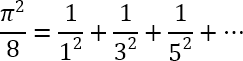7 M
5 (c) Two independent samples of sizes 8 and 7 gave the following results
 Sample 1 : 19 17 15 21 16 18 16 14 Sample 2 : 15 14 15 19 15 18 16
6 M

6 (a) Find the expansion of f(x) = x(π-x), 0 < x < π as a half range cosine series. Hence show that
(i)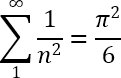(ii)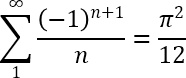7 M
6 (b) The diameter of a semicircular plate of radius a is kept at 0°C and the temperature at the semicircular boundary T°C. Find the steady state temperature u(r,θ)
7 M
6 (c) The average of marks scored by 32 boys is 72 with standard deviation 8 while that of 36 girls is 70 with standard deviation 6. Test at 1% level of significance. Whether the boys perform better than the girls?
6 M

7 (a) Show that the functions f1(x)=1; f2(x)=x are orthogonal on (-1,1). Determine the constants a and b such that the function f3(x)=-1+ax+bx2is orthogonal to both f1 and f2 on that interval.
7 M
7 (b) Find fourier integral represention of
f(x) = x for 0 < x < a
f(x) = 0 for x > a.
and f(-x) = f(x)
7 M
7 (c) If u=x-y, v=x+y and if x,y are uncorrelated, prove that: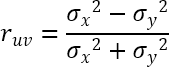6 M

More question papers from Applied Mathematics 4